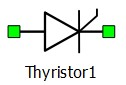# Thyristor

This reference section describes the properties and features of the Thyristor converter component of Typhoon HIL's software.

Important: It is recommended to consider using existing components before incorporating a Thyristor component if possible. Please consult our Knowledgebase article on the recommended uses of the Thyristor component and how to gain access to it.

A block diagram and input parameters for a thyristor are given in Table 1.

Table 1. Thyristor component in Schematic Editor
component component dialog window component parametersThyristor• Use non-ideal switches (if disabled, ideal switch model is used)
• Control source (Digital inputs, Model)
• S1 logic (active high, active low)
• Gate control enabling (enables usage of external digital signal to enable switching)
• Sen (digital input selection for gate enable)
• Sen_logic (active high, active low)
• Snubber type (available if ideal switch is used)
• R (snubber resistance)
• C (snubber capacitance)

Maximal weight = 1

There are two implementations available for the thyristor. It can be modeled using an ideal switch or a non-ideal switch (Figure 1, Figure 2 and Figure 3).

If an ideal switch model is used, thyristor is modeled as a short circuit when conducting and an open circuit when not conducting. In this case, a parallel snubber circuit can be defined. It can be a resistance or a series connection of a resistor and capacitor. These options are shown in Figure 1. If modeled as an ideal switch, the thyristor can be controlled from digital input, or directly from control panel. Weight of thyristor modeled as an ideal switch is 1.Figure 1. R-C snubber option in case of ideal switch model of a diode.

When a Use non-ideal switch option is chosen a thyristor is modeled as a small inductance while conducting and a small capacitances while not-conducting. Weight of thyristor modeled as an ideal switch is 0. Non-ideal switch models are addressing a different resource called Non-ideal switches.The parameter Resistance (Figure 2 and Figure 3) and the simulation step are defining the actual inductance and capacitance values according to the following equations:

$L=Ts\bullet R$

Where L and C are the calculated inductance and capacitance, Ts is the simulation step of electrical part of the model, R is the set resistance value.

Thyristor can be controlled from digital input, model or directly from control panel. Control option can be switched from Digital input (Figure 2) and Model (Figure 3).Figure 2. Property window and symbol of digital input controlled thyristor while modeled as a non-ideal switchFigure 3. Property window and symbol of model controlled thyristor while modeled as a non-ideal switch
Note: Thyristor component is available through special toolbox, which can be provided on request.

## Digital Alias

If a converter is controlled by digital inputs, an alias for every digital input used by the converter will be created. Digital input aliases will be available under the Digital inputs list alongside existing Digital input signals. The alias will be shown as Converter_name.Switch_name, where Converter_name is name of the converter component and Switch_name is name of the controllable switch in the converter.